# 2nd PUC Physics Question Bank Chapter 13 Nuclei

## Karnataka 2nd PUC Physics Question Bank Chapter 13 Nuclei

### 2nd PUC Physics Nuclei NCERT Text Book Questions and Answers

Question 1.
(a) Two stable isotopes of lithium $$_{ 3 }^{ 6 }{ Li\quad }and\quad _{ 3 }^{ 7 }{ Li }$$ have respective abundances of 7.5% and 92.5%. These isotopes have masses of 6.01512 u and 7.01600 u, respectively. Find the atomic mass of lithium.

(b) Boron has two stable isotopes, $$_{ 5 }^{ 10 }{ B\quad }and\quad _{ 5 }^{ 7 }{ B }$$. Their respective masses are 10.01294 u and 11.00931 u, and the atomic mass of boron is 10.811 u. Find the abundances of $$_{ 5 }^{ 11 }{ B\quad }and\quad _{ 5 }^{ 11 }{ B }$$.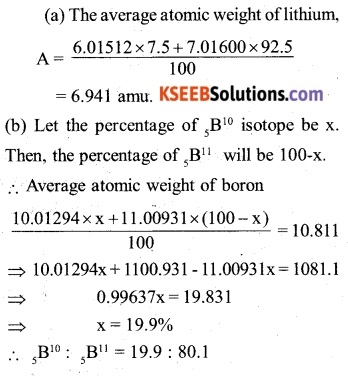Question 2.
The three stable isotopes of neon: $$_{ 10 }^{ 20 }Ne,\quad _{ 10 }^{ 21 }{ Ne }\quad and\quad _{ 10 }^{ 22 }{ Ne }$$ have respective abundances of 90.51%, 0.27% and 9.22%. The atomic masses of the three isotopes are 19.99 u, 20.99 u, and 21.99 u, respectively. Obtain the average atomic mass of neon.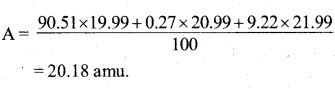Question 3.
Obtain the binding energy (in MeV) of a nitrogen nucleus $$\left( _{ 7 }^{ 14 }{ N } \right)$$ given $$m\left( _{ 7 }^{ 14 }{ N } \right) =14.00307u$$Question 4.
Obtain the binding energy of the nuclei $$_{ 26 }^{ 56 }{ Fe\quad and\quad _{ 83 }^{ 209 }{ Bi } }$$ in units of MeV from the following data:
$$m\left( _{ 26 }^{ 56 }{ Fe } \right) =55.934939u$$
$$m\left( _{ 83 }^{ 209 }{ Bi } \right) =208.980388u$$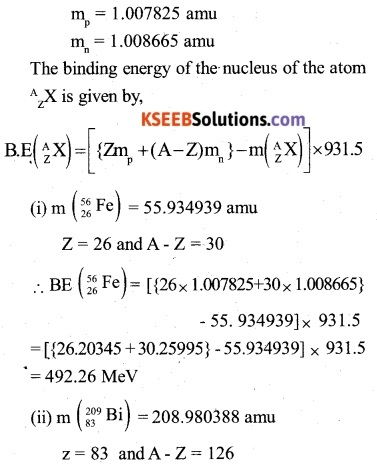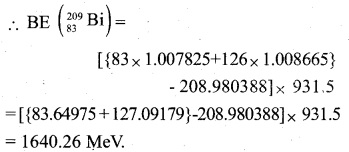Question 5.
A given coin has a mass of 3.0 g. Calculate the nuclear energy that would be required to separate all the neutrons and protons from each other. For simplicity assume that the coin is entirely made of $$_{ 29 }^{ 63 }{ Cu }$$atoms (of mass 62.92960 u).
mp = 1.007825 amu
mn = 1.008665 amu
m (Cu) = 62.92960 amu
The energy required to separate all the neutrons and protons, from each other in the coin is equal to the binding energy of all the atoms in the coin. Number of atoms in the copper coin of mass 3g,The atomic number of copper is 29. Therefore, one atom of copper contains 29 protons and 63-29 i.e. 34 neutrons.
∴ Mass of the constituent nucleons of a copper atom
= 29mp + 34mn
= 29 x 1.007825 + 34 ×1.008665
= 63.521535 amu
∴ Mass defect in a copper atom
= 63.521535-62.92960
= 0.591935 amu
Mass defect of all the atom in the coin,
Δm = 0.591935 x 2.868 x 1022 = 1.698 x 1022 amu
The energy required to separate all the neutrons and protons in the coin,
E = a m x 931.5 = 1.698 x 1022 x 931.5 = 1.582 x 1025 MeV.

Question 6.
Write nuclear reaction equations forQuestion 7.
A radioactive isotope has a half-life of T years. How long will it take the activity to reduce to
(a) 3.125%
(b) 1% of its original value?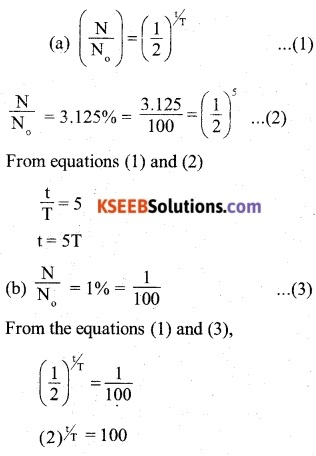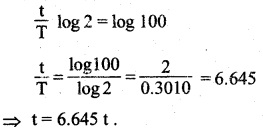Question 8.
The normal activity of living carbon-containing matter is found to be about 15 decays per minute for every gram of carbon. This activity arises from the small proportion of radioactive $$_{ 6 }^{ 14 }C$$ present with the stable carbon isotope $$_{ 6 }^{ 12 }C$$. When the organism is dead, its interaction with the atmosphere (which maintains the above equilibrium activity) ceases and its activity begins to drop. From the known half-life (5730 years) of $$_{ 6 }^{ 14 }C$$ and the measured activity, the age of the specimen can be approximately estimated. This is the principle of $$_{ 6 }^{ 14 }C$$ dating used in archaeo­logy. Suppose a specimen from Mohenjodaro gives an activity of 9 decays per minute per gram of carbon. Estimate the approximate age of the Indus-Valley civilisation.
Suppose that the number of C-14 atoms per gram was No at t = 0 (initially) when its activity was 15 decays per minute per gram. Today, i.e. after time t (age of once-living organism), the number of C-14 atom per gram left is N and it shows an activity of 9 decays per minute per gram. Now, the activity of a radioactive substance is given by,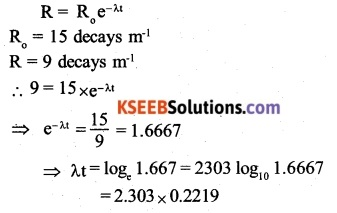Question 9.
Obtain the amount of $$_{ 27 }^{ 60 }Co$$ necessary to provide a radioactive source of 8.0 mCi strength. The half-life of $$_{ 27 }^{ 60 }Co$$ is 5.3 years.Question 10.
The half-life of $$_{ 38 }^{ 90 }Sr$$ is 28 years. What is the disintegration rate of 15 mg of this isotope?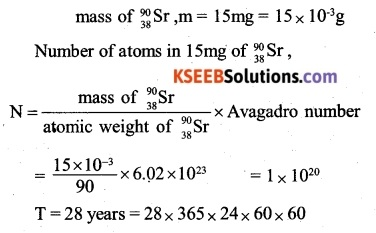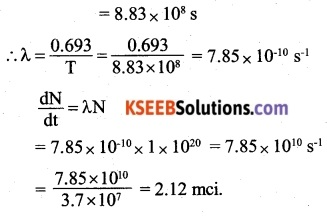Question 11.
Obtain approximately the ratio of the nuclear radii of the gold isotope $$_{ 79 }^{ 197 }{ Au }$$ and the silver $$_{ 49 }^{ 107 }{ Ag }$$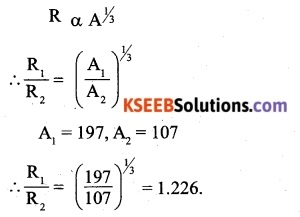Question 12.
Find the Q-value and the kinetic energy of the emitted α -particle in the α -decay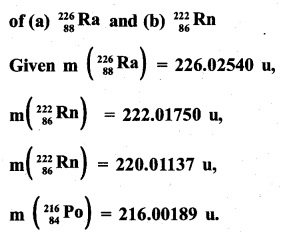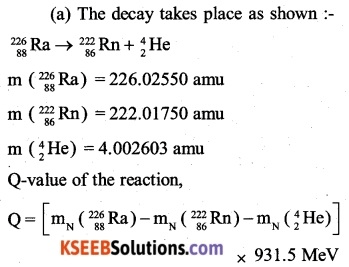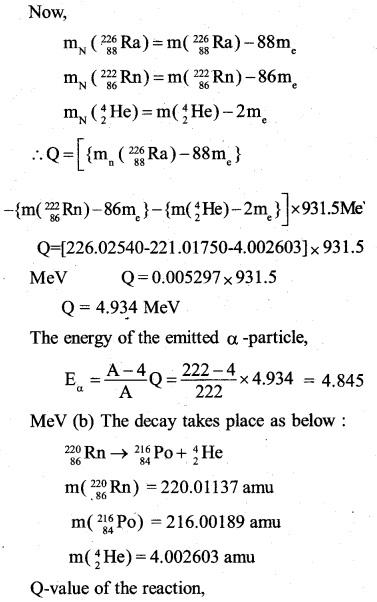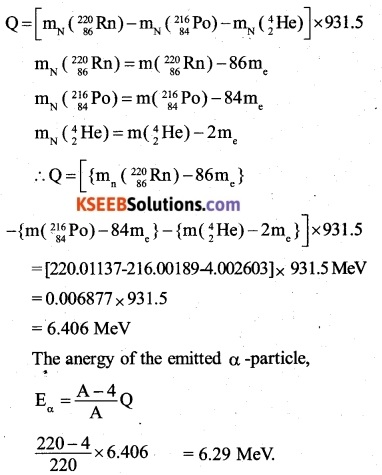Question 13
The radionuclide 11C decays according to $$_{ 6 }^{ 11 }{ C }\rightarrow _{ 5 }^{ 11 }{ B }+{ e }^{ + }+v:{ T }_{ 1/2 }=20.3min$$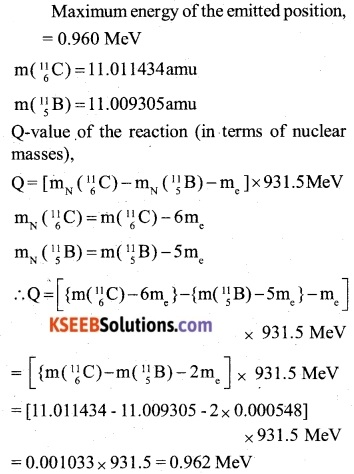The maximum energy of the emitted positron is practically equal to the Q-value of the reaction. It is because the mass of the neutrino is negligibly small as compared to the mass of the positron. Therefore, when they share the energy, the whole of the energy is carried by the positron.Question 14.
The nucleus $$_{ 10 }^{ 23 }{ Ne }$$ decays by β emission. Write down the β -decay equation and determine the maximum kinetic energy of the electrons emitted. Given that: $$_{ 10 }^{ 23 }{ Ne }=22.994466u$$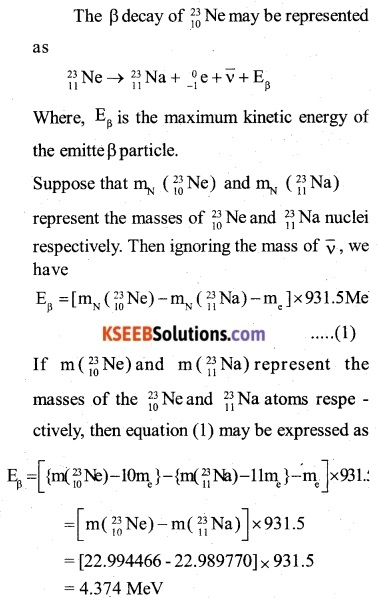The energy released is shared by the Na nucleus and the electron-neutrino pair released. As the electron-neutrino pair is much lighter than the 23 Na nucleus, practically the Whole of the energy released is carried by electron-neutron will carry the maximum energy. Therefore, the maximum kinetic energy of the emitted electron is 4.374 MeV.

Question 15.
The Q value of a nuclear reaction A+b→C+d is defined by Q=[mA+mB-mc-md] where the masses refer to the respective nuclei. Determine from the given data the Q-value of the following reactions and state whether the reactions are exothermic or endothermic.Question 16.
Suppose, we think of fission of a $$_{ 26 }^{ 56 }{ Fe }$$ nucleus into two equal fragments, $$_{ 13 }^{ 28 }{ Al }$$. Is fission energetically possible? Argue by working out Q of the process.
Given m(_{ 26 }^{ 56 }{ Fe })=55.93494u and
$$m(_{ 26 }^{ 56 }{ Al })=27.98191u$$
m(_{ 26 }^{ 56 }{ Fe })=55.93494u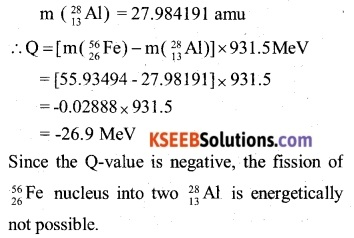Question 17.
The fission properties of $$_{ 94 }^{ 219 }{ Pu }$$ are very similar to those of  $$_{ 92 }^{ 235 }{ U }$$ . The average energy released per fission is 180 MeV. How much energy, in MeV, is released if all the atoms in 1 kg of pure $$_{ 94 }^{ 239 }{ U }$$ undergo fission?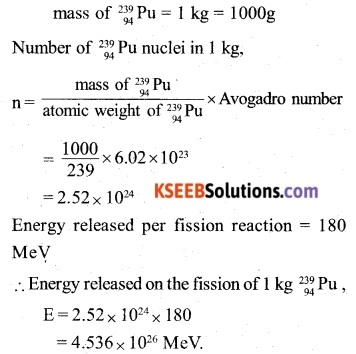Question 18.
A 1000 MW fission reactor consumes half of its fuel in 5.00 y. How much $$_{ 92 }^{ 235 }{ U }$$ did it contain initially? Assume that the reactor operates 80% of the time, that all the energy generated arises from the fission of $$_{ 92 }^{ 235 }{ U }$$ and that this nuclide is consumed only by the fission process.
Power of the reaction, P = 1000 MW = 109W
The reactor operates for 80% of the total time.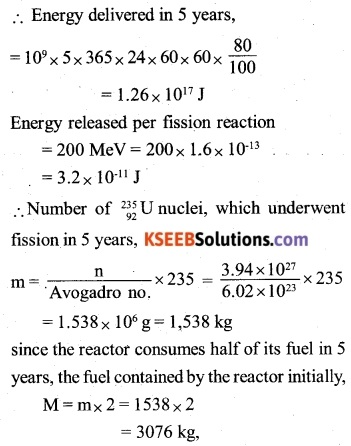Question 19.
How long can an electric lamp of 100W be kept glowing by fusion of 2.0 kg of deuterium? Take the fusion reaction as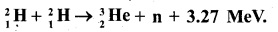Mass of the deuterium, 2 kg = 2000g
Number of deuterium nuclei in 2 kg,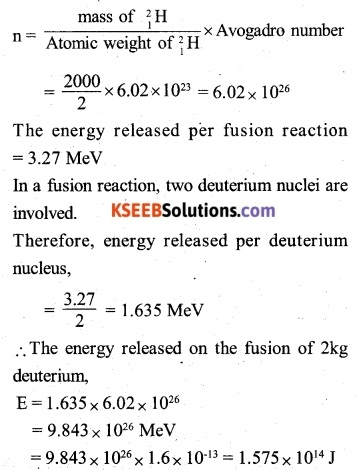Question 20.
Calculate the height of the potential barrier for a head-on collision of two deuterons. (Hint: The height of the potential barrier is given by the Coulomb repulsion between the two deuterons when they just touch each other. Assume that they can be taken as hard spheres of radius 2.0 fm.)
In the head-on collision of two deuterons, the two nuclei come to close up to a distance equal to the sum of their radii
∴r = 2 + 2=4fm = 4×10-15m
The charge on a deuteron
e = charge on a proton = 1.6x 10-19c
∴ The potential energy of the deuterons during a head-on collision,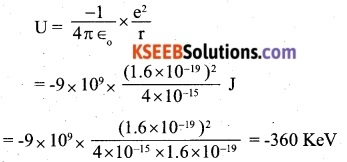∴ A deuteron will require a Kinetic energy of 360 keV to surmount the potential barrier.

Question 21.
From the relation $$R={ R }_{ 0 }{ A }^{ 1/2 }$$ where R0 is a constant and A is the mass number of a nucleus, show that the nuclear matter density is nearly constant. (i.e. independent of A).
Let us find the density of the nucleus of an atom, whose mass number is A.
The mass of the nucleus of the atom of mass number AQuestion 22.
For the β+ (positron) emission from a nucleus, there is another competing process known as electron capture (electron from an inner orbit, say, the K- shell, is captured by the nucleus and a neutrino is emitted).
$${ e }^{ + }+_{ z }^{ A }{ X }\rightarrow _{ Z-1 }^{ A }{ Y+v }$$
Show that if β+ emission is energetically allowed, electron capture is necessarily allowed but not vice-versa.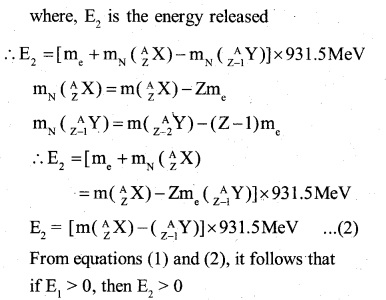From equations (1) and (2), it follows that if E1> 0, then E2 > 0
i.e if β+emission is energetically allowed, then the electron capture is necessarily allowed. On the other hand, if E2 > 0, then it does not necessarily mean that E2> 0, Thus if the electron capture is allowed, then β+emission is not necessarily allowed.

### 2nd PUC Physics Nuclei Additional Exercises

Question 23.
In a periodic table the average atomic. mass of magnesium is given as 24.312 u. The average value is based on their relative natural abundance on earth. The three isotopes and their masses are $$_{ 12 }^{ 24 }{ Mg }(23.98504u)$$, $$_{ 12 }^{ 24 }{ Mg }(24.98584u)$$ and $$_{ 12 }^{ 24 }{ Mg }(25.98259u)$$. The natural abundance of $$_{ 12 }^{ 24 }{ Mg }\quad is\quad 78.99%$$ by mass. Calculate the abundances of other two isotopes.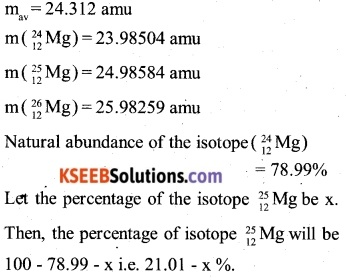Question 24.
The neutron separation energy is defined as the energy required to remove a neutron from the nucleus. Obtain the neutron separation energies of the nuclei $$_{ 20 }^{ 41 }{ Ca }_{ 13 }^{ 27 }{ Al }$$ from the following data:
$$m\left( _{ 20 }^{ 40 }{ Ca } \right) =39.962591u$$
$$m\left( _{ 20 }^{ 41 }{ Ca } \right) =40.962278u$$
$$m\left( _{ 13 }^{ 26 }Al \right) =25.986895u$$
$$m\left( _{ 13 }^{ 27 }Al \right) =26.981541u$$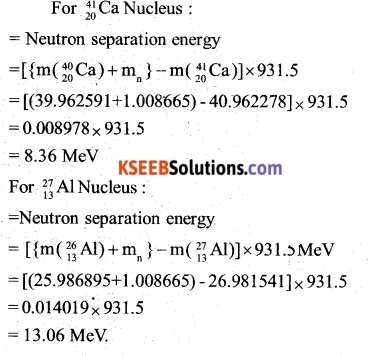Question 25.
A source contains two phosphorous radio nuclides $$_{ 15 }^{ 33 }{ P({ T }_{ 1/2 }=14.3d) }\quad and\quad _{ 15 }^{ 33 }{ P }{ ({ T }_{ 1/2 }=25.3d) }$$Initially, 10% of the decays come from $${ { T }_{ 1/2 } }$$. How long one must wait until 90% do so?
Initially 10% of the decays come from $$_{ 15 }^{ 33 }{ P }$$ the number of nuclei of $$_{ 15 }^{ 33 }{ P }$$ left behind will be 90% and that of $$_{ 15 }^{ 33 }{ P }$$ will be 10%. Let N, and N2 be the number of the nuclei of $$_{ 15 }^{ 33 }{ P }$$ and $$_{ 15 }^{ 33 }{ P }$$ present initially. It follows that  if N, = 9N1, then N2 = N
suppose that 90% of $$_{ 15 }^{ 33 }{ P }$$ nuclei decay in time t. Let N’1 and N’2 be the numbers of the nuclei of $$_{ 15 }^{ 33 }{ P }$$ and $$_{ 15 }^{ 33 }{ P }$$ present after the time t. It follows that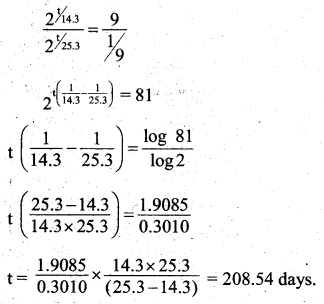Question 26.
Under certain circumstances, a nucleus can decay by emitting a -particle more massive than an a-particle. Consider the following decay processes: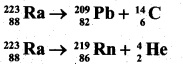Calculate the Q-values for these decays and determine that both are energetically allowed.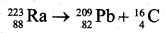Question 27.
Consider the fission of $${ _{ 92 }^{ 238 }{ U } }$$ by fast neutrons. In one fission event, no neutrons are emitted and the final end products, after the beta decay of the primary fragments, are $${ _{ 58 }^{ 140 }{ Ce } }\quad and\quad _{ 44 }^{ 99 }{ Ru }$$. Calculate Q for this fission process. The relevant atomic and particle masses areQuestion 28.
Consider the D-T reaction (deuterium-tritium fusion)
$${ _{ 1 }^{ 2 }{ Ce } }\quad +\quad _{ 1 }^{ 3 }{ Ru\rightarrow _{ 2 }^{ 4 }{ He }+n }$$
(a) Calculate the energy released in MeV in this reaction from the data:
$$m(_{ 1 }^{ 2 }{ H })=2.014102u$$
$$m(_{ 1 }^{ 3 }{ H })=3.016049u$$
(b) Consider the radius of both deuterium and tritium to be approximately 2.0 fm. What is the kinetic energy needed to overcome the coulomb repulsion between the two nuclei? To what temperature must the gas be heated to initiate the reaction? (Hint: Kinetic energy required for one fusion event =average thermal kinetic energy available with the interacting particles = 2(3kT/2); k = Boltzman’s constant!, T = absolute temperature.)the two nuclei almost come in contact with other is given by,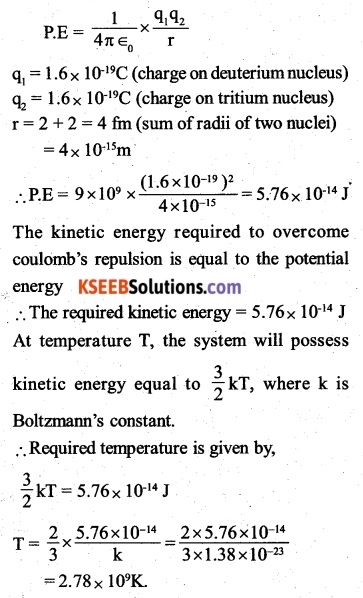Question 29.
Obtain the maximum kinetic energy of β-particles, and the radiation frequencies of γ decays in the decay scheme shown in Fig. 13.6. You are given that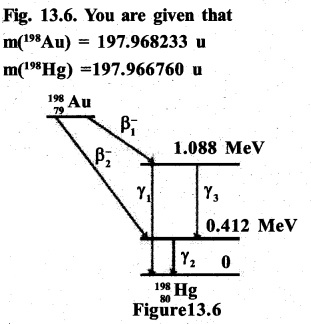E1=0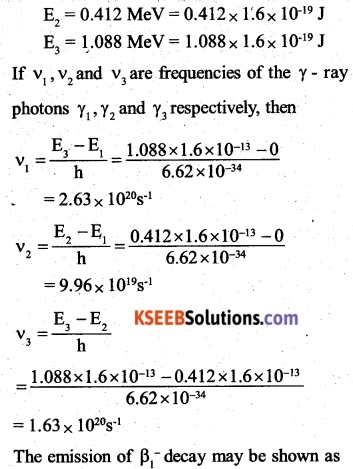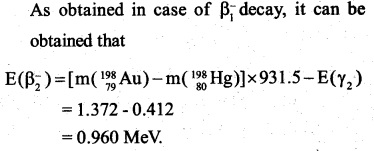Question 30.
Calculate and compare the energy released by a) fusion of 1.0 kg of hydrogen deep within Sun and b) the fission of 1.0 kg of 235U in a fission reactor.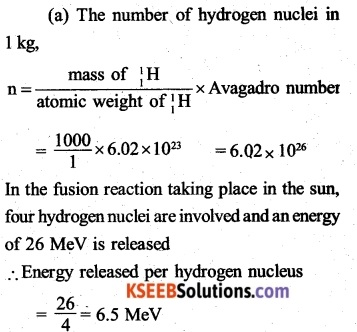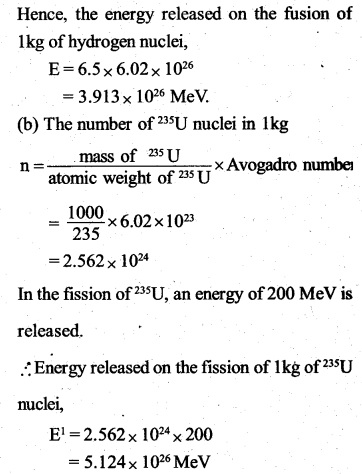Question 31.
Suppose India had a target of producing by 2020 AD, 200,000 MW of electric power, ten percent of which was to be obtained from nuclear power plants. Suppose we are given that, on average, the efficiency of utilization (i.e. conversion to electric energy) of thermal energy produced in a reactor was 25%. How much amount of fissionable uranium would our country need per year by 2020? Take the heat energy per fission of 235U to be about 200MeV,
Energy released per fission = 200 MeV
Efficiency of utilization = 25%
∴ Electrical energy produced per fissionQuestion 1.
Two nuclei have mass numbers in the ratio 1:2, what is the ratio of the nuclear densities?
Nuclear density is independent of the mass number of a nucleus. Since all nuclei have same nuclear density (approximately), their ratio of nuclear densities is 1:1

Question 2.
Calculate the density of hydrogen nucleus in SI units R0 = 1.1 x10-15 m and  a.m.u = 66 x 1027 kg.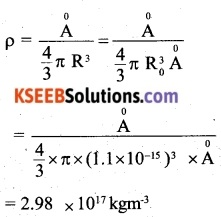Question 3.
The half-life period of a radioactive substance is 30 days. What is the time taken for $$\frac { { 3 }^{ th } }{ 4 }$$ of its original mass to
disintegrate?Question 4.
Half-life, T = 138 days
Half-life of a certain radioactive material is 138 days. After what lapse of time, the undecayed- fraction of the material will be 12.5%?Question 5.
The half life of $$_{ 92 }^{ 238 }{ U }$$ against decay is 4.5 x 109 years. How many disintegrations per second occur in 1 kg of $$_{ 92 }^{ 238 }{ U }$$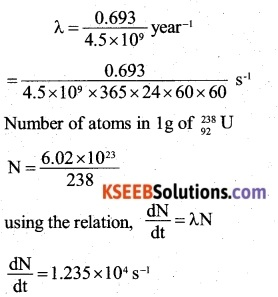Question 6.
Which of the following cannot be emitted by radioactive substance during their decay?
(A) Protons
(B) Neutrinos
(C) Helium nuclei
(D) Electrons
(A) Protons

Question 7.
Starting with a sample of pure $$^{ 66 }{ Cu,\frac { 7 }{ 8 } }$$ of it decays into Zn in 15 minutes. The corresponding half life is
(A) 10 minutes
(B) 15 minutes
(C) 5 minutes
(D) 7.5 minutes
The undecayed mass left after time t is given by,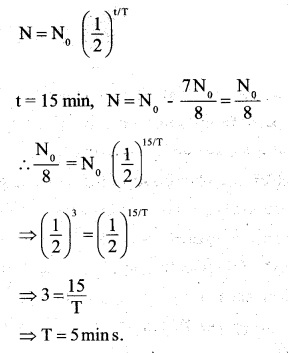Question 8.
In r-ray emission from a nucleus:
(A) Both neutron number and proton number change
(B) There is no change in neutron and proton number
(C) Only the neutron number changes
(D) only the proton number changes
(B) There is no change in neutron and proton numberQuestion 9.
If the binding energy per nucleon in  $$_{ 3 }^{ 4 }{ Li }\quad and\quad _{ 3 }^{ 4 }{ He }$$ nuclei are 5.60 MeV and 7.06 MeV respectively, then in reaction $$_{ 1 }^{ 1 }{ Li }+_{ 3 }^{ 4 }{ He }\rightarrow 2\quad _{ 2 }^{ 4 }{ He }$$ energy of proton must be
(A) 28.24 MeV
(B) 17.28 MeV
(C) 1.46 MeV
(D) 39.2 MeV